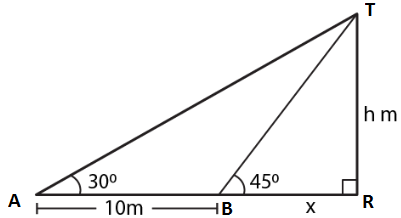Guru

# From the top of a building 20 m high, the angle of elevation of the top of a monument is 45 and the angle of depression of its foot is 15. Find the height of the monument.

• 0

sir this is the important  question from the book -ML aggarwal( avichal publication) class 10th , chapter20 , heights and distances
it is given that From the top of a building 20 m high,

the angle of elevation of the top of a monument is 45 and the angle of depression of its foot is 15. Find the height of the monument.

question no 35 , heights and distances , ICSE board, ML Aggarwal

Share

1. Consider TR as the tower where TR = h

BR = x

AB = 10 m

Angles of elevation from the top of the tower at A and B are 300 and 450In right triangle TAR

tan θ = TR/AR

Substituting the values

tan 300 = h/ (10 + x)

So we get

1/√3 = h/ (10 + x)

h = (10 + x)/ √3 …. (1)

In triangle TBR

tan 450 = TR/BR = h/x

So we get

1 = h/x

x = h ….. (2)

Using both the equations

h = (10 + h)/ √3

√3h = 10 + h

By further calculation

√3h – h = 10

(1.732 – 1) h = 10

0.732 h = 10

h = 10/0.732 = 13.66

Hence, the height of the tower is 13.7 m.

• 0